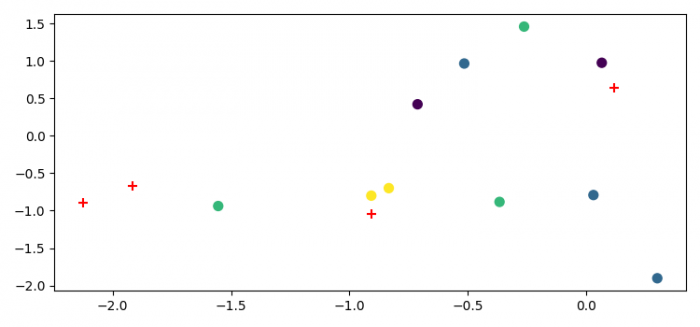# How to make a scatter plot for clustering in Python?

To make a scatter plot for clustering in Python, we can take the following steps −

• Set the figure size and adjust the padding between and around the subplots.
• Create x and y data points, Cluster and centers using numpy.
• Create a new figure or activate an existing figure.
• Add a subplot arrangement to the current figure.
• Plot the scatter data points using scatter() method.
• Iterate centers data and place marker using scatter() method.
• To display the figure, use show() method.

## Example

import numpy as np
import matplotlib.pyplot as plt

plt.rcParams["figure.figsize"] = [7.00, 3.50]
plt.rcParams["figure.autolayout"] = True

x = np.random.randn(10)
y = np.random.randn(10)
Cluster = np.array([0, 1, 1, 1, 3, 2, 2, 3, 0, 2])
centers = np.random.randn(4, 2)

fig = plt.figure()

scatter = ax.scatter(x, y, c=Cluster, s=50)
for i, j in centers:
ax.scatter(i, j, s=50, c='red', marker='+')

plt.show()

## Output

It will produce the following output